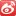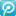# python计算字符串相似度_字符串相似度算法Python实现

#!/usr/bin/env python

def levenshtein(a,b):

"Calculates the Levenshtein distance between a and b."

n, m = len(a), len(b)

if n > m:

# Make sure n <= m, to use O(min(n,m)) space

a,b = b,a

n,m = m,n

current = range(n+1)

for i in range(1,m+1):

previous, current = current, [i]+*n

for j in range(1,n+1):

change = previous[j-1]

if a[j-1] != b[i-1]:

change = change + 1

return current[n]

def levenshtein_distance(first, second):

"""Find the Levenshtein distance between two strings."""

if len(first) > len(second):

first, second = second, first

if len(second) == 0:

return len(first)

first_length = len(first) + 1

second_length = len(second) + 1

distance_matrix = [range(second_length) for x in range(first_length)]

for i in range(1, first_length):

for j in range(1, second_length):

deletion = distance_matrix[i-1][j] + 1

insertion = distance_matrix[i][j-1] + 1

substitution = distance_matrix[i-1][j-1]

if first[i-1] != second[j-1]:

substitution += 1

distance_matrix[i][j] = min(insertion, deletion, substitution)

return distance_matrix[first_length-1][second_length-1]

if __name__=="__main__":

#from sys import argv

first = "abcabcaa"

second = "cbsaaecaa"

print levenshtein(first,second)

print levenshtein_distance(first,second)2009-02-05 16:33

03-26775

03-2681
01-191万+
05-071万+
03-261444
11-21804
12-151万+
02-012011
06-21559
08-191268
09-108006
03-31199
02-281万+
08-051万+
12-062208
05-187178
10-161万+

### “相关推荐”对你有帮助么？

•非常没帮助
•没帮助
•一般
•有帮助
•非常有帮助¥2 ¥4 ¥6 ¥10 ¥20余额支付 (余额：-- )扫码支付获取中扫码支付点击重新获取扫码支付1.余额是钱包充值的虚拟货币，按照1:1的比例进行支付金额的抵扣。
2.余额无法直接购买下载，可以购买VIP、C币套餐、付费专栏及课程。余额充值